• +91 9971497814
• info@interviewmaterial.com

### Related Subjects

Question 1 : A piece of cloth costs Rs. 35. If the piece were 4 m longer and each metre costs Re. one less, the cost would remain unchanged. How long is the piece ?

Let the length of piece of cloth = x m
Total cost = Rs. 35
Cost of 1 m cloth = Rs. 35/x
According to the condition,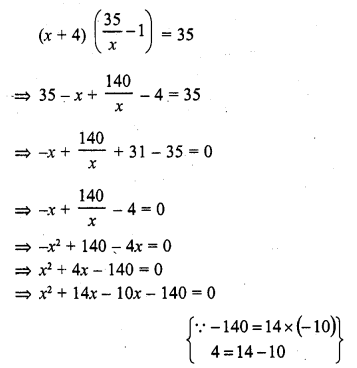=> x (x + 14) – 10 (x + 14) = 0
=> (x + 14) (x – 10) = 0
Either x + 14 = 0, then x = – 14 which is not possible being negative
or x – 10 = 0, then x = 10
Length of piece of cloth = 10 m

Question 2 : Some students planned a picnic. The budget for food was Rs. 480. But eight of these failed to go and thus the cost of food for each member increased by Rs. 10. How many students attended the picnic ?

Let the number of students = x
and total budget = Rs. 480
Share of each students = Rs. 480/x
According to the condition,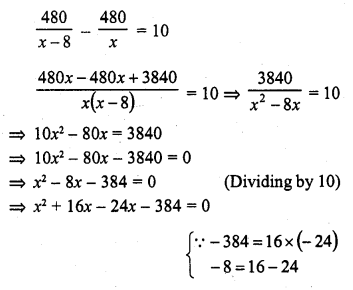=> x (x + 16) – 24 (x + 16) = 0
=> (x + 16) (x – 24) = 0
Either x + 16 = 0, then x = -16 which is not possible being negative
or x – 24 = 0, then x = 24
Number of students = 24
and number of students who attended the picnic = 24 – 8 = 16

Question 3 : A dealer sells an article for Rs. 24 and gains as much percent as the cost price of the article. Find the cost price of the article.

Let cost price ofjfie article = Rs. x
Selling price = Rs. 24
Gain = x %
According to the condition,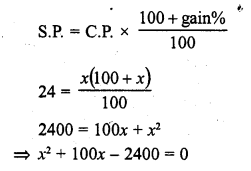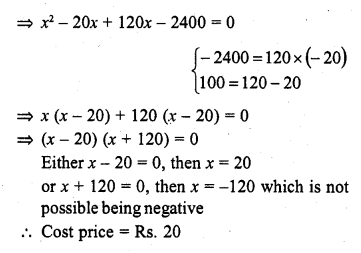Question 4 : Out of a group of swans, 7/2 times the square root of the total number are playing on the share of a pond. The two remaining ones are swinging in water. Find the total number of swans.

Let the total number of swans = x
According to the condition,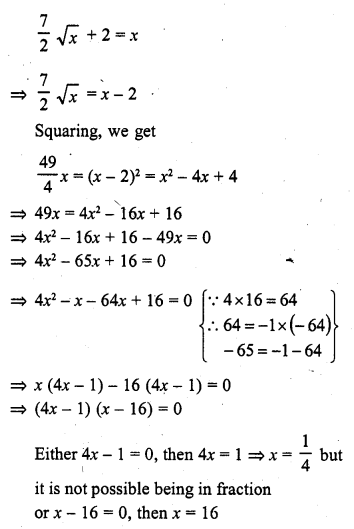Number of total swans = 16

Question 5 : If the list price of a toy is reduced by Rs. 2, a person can buy 2 toys mope for Rs. 360. Find the original price of the toy. (C.B.S.E. 2002C)

List price of the toy = Rs. x
Total amount = Rs. 360
Reduced price of each toy = (x – 2)
According to the condition,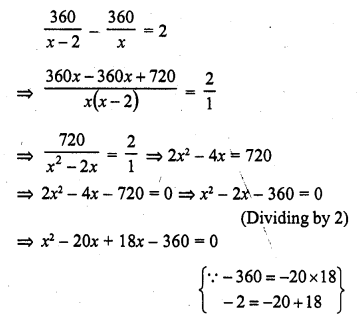=> x (x – 20) + 18 (x – 20) = 0
=> (x – 20) (x + 18) = 0
Either x – 20 = 0, then x = 20
or x + 18 = 0, then x = -18 which is not possible being negative
Price of each toy = Rs. 20

Question 6 : Rs. 9000 were divided equally among a certain number of persons. Had there been 20 more persons, each would have got Rs. 160 less. Find the original number of persons.

Total amount = Rs. 9000
Let number of persons = x
Then each share = Rs. 9000/x
Increased persons = (x + 20)
According to the condition,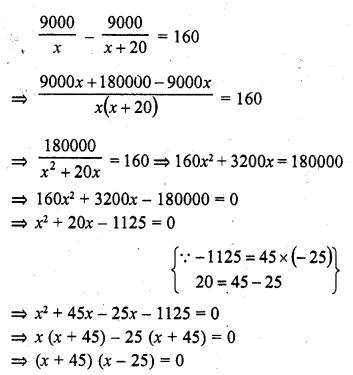Either x + 45 = 0, then x = -45 which is not possible being negative
or x – 25 = 0, then x = 25
Number of persons = 25

Question 7 : Some students planned a picnic. The budget for food was Rs. 500. But 5 of them failed to go and thus the cost of food for each number increased by Rs. 5. How many students attended the picnic? (C.B.S.E. 1999)

Let number of students = x
Total budget = Rs. 500
Share of each student = Rs. 500/x
No. of students failed to go = 5
According to the given condition,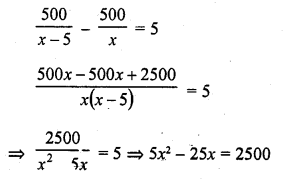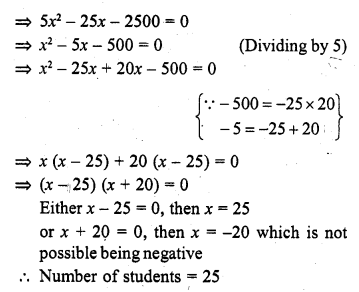Question 8 : A pole has to be erected at a point on the boundary of a. circular park of diameter 13 metres in such a way that the difference, of its distances from two diametrically opposite fixed gates A and B on the boundary is 7 metres. Is it the possible to do so ? If yes, at what distances from the two gates Should the pole be erected ?

In a circle, AB is the diameters and AB = 13 m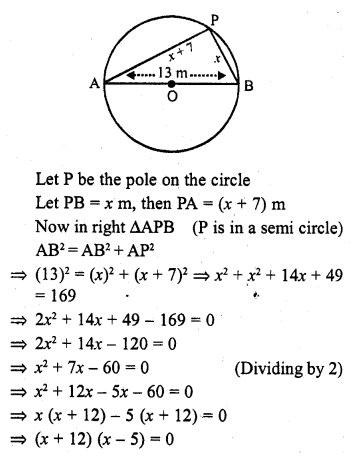Either x + 12 = 0, then x = -12 which is not possible being negative
or x – 5 = 0, then x = 5
P is at a distance of 5 m from B and 5 + 7 = 12 m from A

Question 9 : In a class test, the sum of the marks obtained by P in Mathematics and Science is 28. Had he got 3 marks more in Mathematics and 4 marks less in Science. The product of their marks, would have been 180. Find his marks in the two subjects. (C.B.S.E. 2008)

Sum of marks in Mathematics and Science = 28
Let marks in Math = x
Then marks in Science = 28 – x
According to the condition,
(x + 3) (28 – x – 4) = 180
=> (x + 3) (24 – x) = 180
=> 24x – x² + 72 – 3x = 180
=> 21x – x² + 72 – 180 = 0
=> – x² + 21x – 108 = 0
=> x² – 21x + 108 = 0
=> x² – 9x – 12x + 108 = 0
=> x (x – 9) – 12 (x – 9) – 0
=> (x – 9)(x – 12) = 0
Either x – 9 = 0, then x = 9
or x – 12 = 0, then x = 12
(i) If x = 9, then Marks in Maths = 9 and marks in Science = 28 – 9 = 19
(ii) If x = 12, then Marks in Maths = 12 and marks in Science = 28 – 12 = 16

Question 10 : In a class test, the sum of Shefali’s marks in Mathematics and English is 30. Had she got 2 marks more in Mathematics and 3 marks less in English, the product of her marks would have been 210. Find her marks in two subjects. [NCERT]

Sum of marks in Mathematics and English = 30
Let marks obtained in Mathematics = x
Then in English = 30 – x
According to the condition,
(x + 2) (30 – x – 3) = 210
=> (x + 2) (27 – x) = 210
=> 27x – x² + 54 – 2x – 210 = 0
=> – x² + 25x – 156 = 0
=> x² – 25x + 156 = 0
=> x² – 12x – 13x +156 = 0
=> x (x – 12) – 13 (x – 12) = 0
=> (x – 12) (x – 13) = 0
Either x – 12 = 0, then x = 12
or x – 13 = 0, then x = 13
(i) If x = 12, then
Marks in Maths =12 and in English = 30 – 12 = 18
(ii) If x = 13, then
Marks in Maths = 13 and in English = 30 – 13 = 17

Todays Deals### RD Chapter 8- Quadratic Equations Ex-8.13 Contributorskrishan

Name:
Email:

# Latest News# 9000 interview questions in different categories